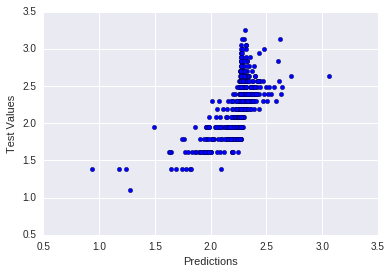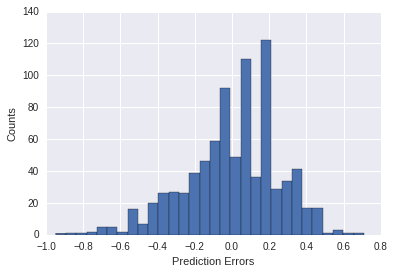# Scikit-learn Basics Tutorial¶

Abalones are marine snails:There is a somewhat well-known dataset involving abalones that is commonly use to test machine learning algorithms. In this Jupyter notebook we'll see how to use scikit-learn to test a few models against this dataset.

Abalones have some easily measurable features, such as their physical dimensions and weight, and some difficult to measure features, such as their age. To determine the age, the abalone must be stained and its growth rings counted under a microscope, which is clearly a tedious and time-consuming task.

This dataset is commonly used to test out machine learning algorithms and has a reputation of being difficult to achieve a good score on, so our main goal will be just to demonstrate the use of scikit-learn and pandas in a Jupyter notebook (download this notebook). You can find a somewhat similar modeling exercise using R here.

In :
# Suppress warnings
import warnings
warnings.filterwarnings('ignore')

In :
import matplotlib.pyplot as plt
import numpy as np
import pandas as pd
import seaborn as sns

%matplotlib inline

# Read the data into a pandas dataframe
# We need to set the column names manually because they are not in
# the data file

names = ["Sex", "Length", "Diameter", "Height", "Whole weight", "Shucked weight",
"Viscera weight", "Shell weight", "Rings"]


Out:
Sex Length Diameter Height Whole weight Shucked weight Viscera weight Shell weight Rings
0 M 0.455 0.365 0.095 0.5140 0.2245 0.1010 0.150 15
1 M 0.350 0.265 0.090 0.2255 0.0995 0.0485 0.070 7
2 F 0.530 0.420 0.135 0.6770 0.2565 0.1415 0.210 9
3 M 0.440 0.365 0.125 0.5160 0.2155 0.1140 0.155 10
4 I 0.330 0.255 0.080 0.2050 0.0895 0.0395 0.055 7

# Exploratory Analysis¶

First let's take a look at the data. There are a number of physical measurements of size that may be correlated.

In :
vars=["Length", "Diameter", "Height", "Rings"]
sns.pairplot(df, vars=vars, hue="Sex")

Out:
<seaborn.axisgrid.PairGrid at 0x7f18d40b4d68>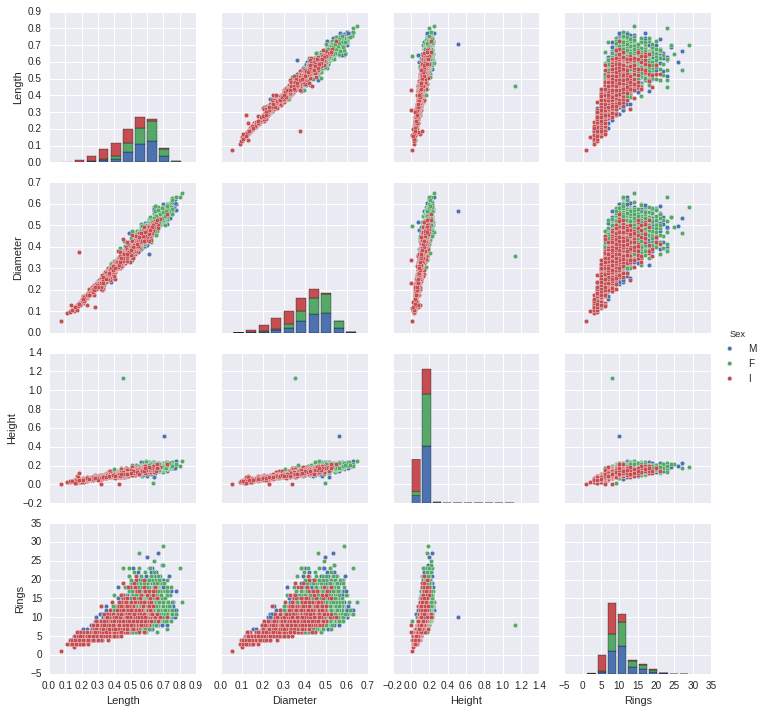The data also includes a number of weight measurements that may be correlated.

In :
vars=["Whole weight", "Shucked weight", "Viscera weight",
"Shell weight", "Rings"]
sns.pairplot(df, vars=vars, hue="Sex")

Out:
<seaborn.axisgrid.PairGrid at 0x7f18a66d4ba8>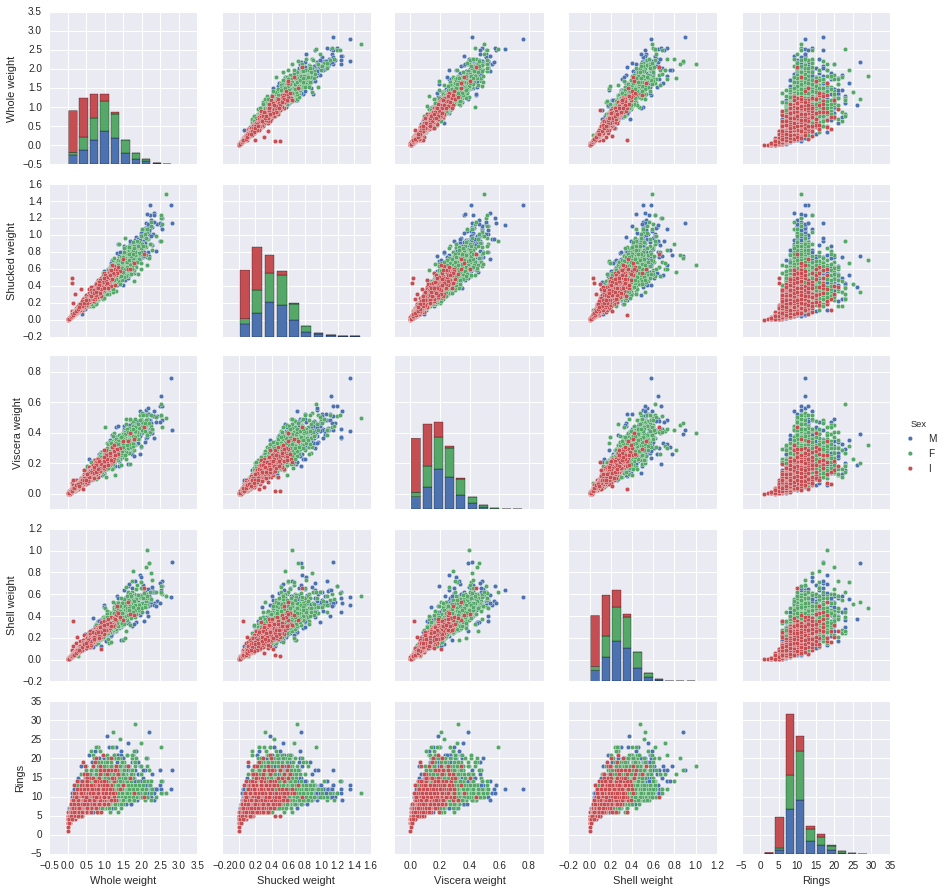The data also includes a label indicating the sex of the abalone (if an adult), with the value I if not yet an adult.

In :
g = sns.FacetGrid(df, col="Sex", margin_titles=True)
g.map(sns.regplot, "Whole weight", "Rings",
fit_reg=False, x_jitter=.1);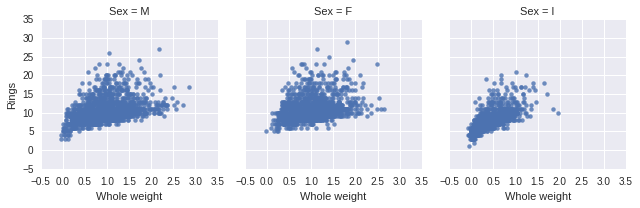From the exploratory analysis we can see a few obvious relationships:

• The weight variables are highly correlated
• Sex significantly changes the relationship between weight and age (Rings)
• The relationship between weight and Rings is not linear
• Length, Height, and Diameter are all fairly correlated

# Fitting Models¶

Our task is to develop a model to determine the age of the abalone that does not require an expensive direct measurement (manual staining and counting growth rings). It's easier to measure the weight, sex, and physical dimensions than the number of rings, so if we can predict the age accurately from easy measurables we can eliminate the difficult age determination.

We can try to predict the age (Rings) either as a continuous variable or as a discrete category. Let's treat the Rings variable as a label and try to predict labels from the other data. Referencing the scikit-learn algorithm flowchart, it looks like a linear SVC is a goodfirst choice.

We need to map the sex to an integer variable to make scikit-learn happy. We'll also need to split the data into testing and training subsets.

In :
# Map Sex to integers
mapping = dict(zip(["I", "F", "M"], [0, 1, 2]))
df.replace({"Sex": mapping}, inplace=True)

Out:
Sex Length Diameter Height Whole weight Shucked weight Viscera weight Shell weight Rings
0 2 0.455 0.365 0.095 0.5140 0.2245 0.1010 0.150 15
1 2 0.350 0.265 0.090 0.2255 0.0995 0.0485 0.070 7
2 1 0.530 0.420 0.135 0.6770 0.2565 0.1415 0.210 9
3 2 0.440 0.365 0.125 0.5160 0.2155 0.1140 0.155 10
4 0 0.330 0.255 0.080 0.2050 0.0895 0.0395 0.055 7
In :
# Split off the feature to predict
targets = df["Rings"]
del df["Rings"]

In :
# Scale the data since SVMs aren't scale invariant
from sklearn import preprocessing
df = pd.DataFrame(preprocessing.scale(df),
index = df.index, columns = df.columns)

# Split our data into training and testing
from sklearn.cross_validation import train_test_split
X_train, X_test, y_train, y_test = train_test_split(df, targets,
test_size = 0.2)


Since we will want to compare multiple classifiers, let's write a short convenience function to test a classifier and display the results.

In :
def try_classifier(classifier, X_train, X_test, y_train, y_test):
# Train the classifier
classifier.fit(X_train, y_train)

# Generate predictions
predictions = classifier.predict(X_test)

# Plot the predictions versus the true values
plt.scatter(predictions, y_test)
plt.ylabel("Test Values")
plt.xlabel("Predictions")

# Plot histogram of errors
plt.figure()
_ = plt.hist(predictions - y_test, bins=30)
plt.ylabel("Counts")
plt.xlabel("Prediction Errors")

# Report the goodness of fit
print("Classifier Score:", classifier.score(X_test, y_test))


Ok, now we're ready to try a simple model on the data.

In :
from sklearn.svm import LinearSVC

classifier = LinearSVC()
try_classifier(classifier, X_train, X_test, y_train, y_test)

Classifier Score: 0.275119617225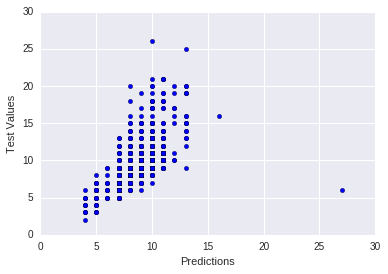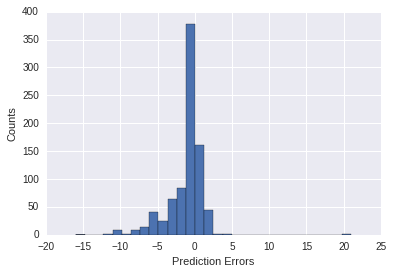Not the best fit ever. Let's try a different kernel.

In :
from sklearn.svm import SVC

classifier = SVC(kernel="rbf")
try_classifier(classifier, X_train, X_test, y_train, y_test)

Classifier Score: 0.278708133971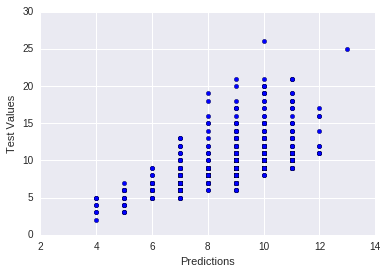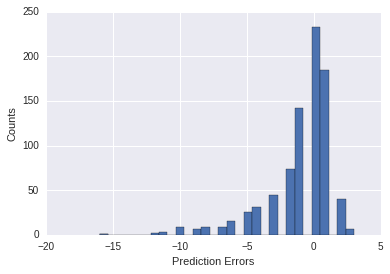Not much improvement from the rbf kernel. We can try to tune the hyperparameters with cross-validation (this might take a while longer).

In :
from sklearn.cross_validation import ShuffleSplit
from sklearn.grid_search import GridSearchCV

estimator = SVC(kernel="linear")
cv = ShuffleSplit(X_train.shape, n_iter=10, test_size=0.2,
random_state=0)
gammas = np.logspace(-10, -1, 10)
classifier = GridSearchCV(estimator=estimator, cv=cv,
param_grid=dict(gamma=gammas))
try_classifier(classifier, X_train, X_test, y_train, y_test)

Classifier Score: 0.283492822967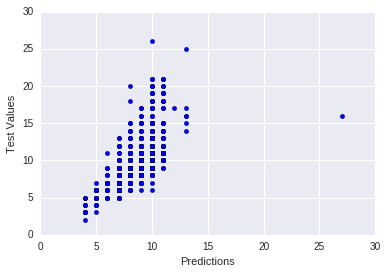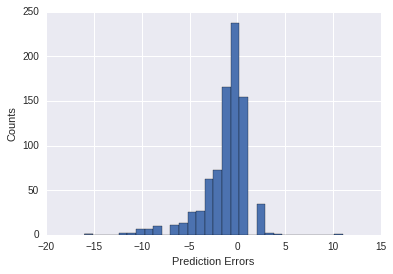Let's try a different classifying algorithm for comparison, a random forest model.

In :
from sklearn.ensemble import RandomForestClassifier
classifier = RandomForestClassifier(max_depth=6, n_estimators=40,
max_features=8)

try_classifier(classifier, X_train, X_test, y_train, y_test)

Classifier Score: 0.265550239234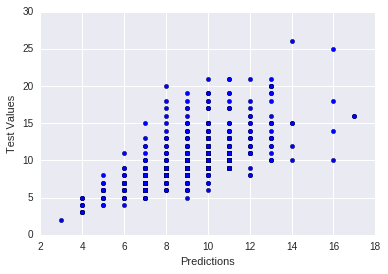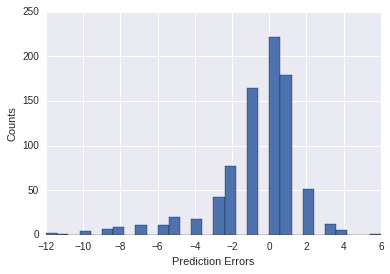Instead of treating the number of rings as a label, let's try to quantitatively predict the number of Rings with a different type of model. In this case a generalized linear model or lasso is a reasonable place to start. Here our classifiers are really estimators, but I'll stick with a consistant notation.

In :
from sklearn import linear_model
classifier = linear_model.Lasso(alpha=0.1)

targets = np.log(targets)
X_train, X_test, y_train, y_test = train_test_split(df, targets,
test_size = 0.2)

try_classifier(classifier, X_train, X_test, y_train, y_test)

Classifier Score: 0.345093281198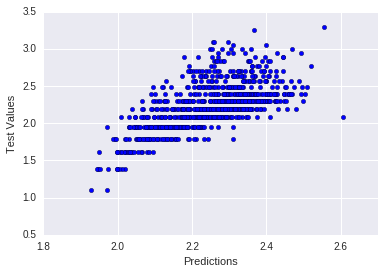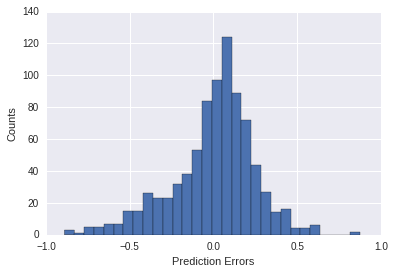While not a great model, this dataset has a reputation for being difficult to model, and this is our best guess yet.

# Feature Selection¶

Since we know some of the variables are highly correlated, we could attempt to simplify the model using feature selection. In this case we could probably simply guess the features, likely Sex, Whole Weight, Height, and Diameter.

In :
# Recall the names
names = ["Sex", "Length", "Diameter", "Height", "Whole weight",
"Shucked weight", "Viscera weight", "Shell weight", "Rings"]

df2 = df[["Sex", "Diameter", "Height", "Whole weight"]]

X_train, X_test, y_train, y_test = train_test_split(df2, targets,
test_size = 0.2)

classifier = linear_model.Lasso(alpha=0.1)

classifier.fit(X_train, y_train)

try_classifier(classifier, X_train, X_test, y_train, y_test)

Classifier Score: 0.335993686985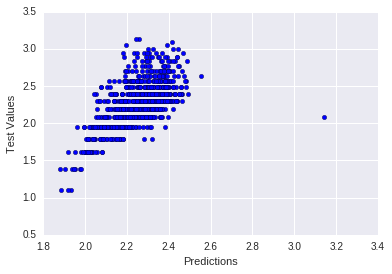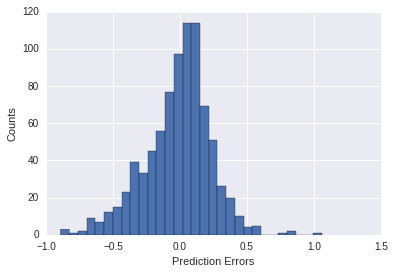As we can see, this reduced set of features achieves about the same level of accuracy. We could also try to derive new features from the data. For example, we could treat the snail as an ellipsoid and compute the volume (proportional to $l * w * h$) for use in model development.

In :
df["Volume"] = df[["Length", "Diameter", "Height"]].product(axis=1)

df2 = df[["Sex", "Volume", "Whole weight"]]

X_train, X_test, y_train, y_test = train_test_split(df2, targets,
test_size = 0.2)

classifier = linear_model.Lasso(alpha=0.1)

classifier.fit(X_train, y_train)

try_classifier(classifier, X_train, X_test, y_train, y_test)

Classifier Score: 0.340433331772